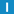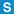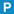# class DateTime

Library: Foundation
Package: DateTime

## Description

This class represents an instant in time, expressed in years, months, days, hours, minutes, seconds and milliseconds based on the Gregorian calendar. The class is mainly useful for conversions between UTC, Julian day and Gregorian calendar dates.

The date and time stored in a DateTime is always in UTC (Coordinated Universal Time) and thus independent of the timezone in effect on the system.

Conversion calculations are based on algorithms collected and described by Peter Baum at http://vsg.cape.com/~pbaum/date/date0.htm

Internally, this class stores a date/time in two forms (UTC and broken down) for performance reasons. Only use this class for conversions between date/time representations. Use the Timestamp class for everything else.

Notes:

• Zero is a valid year (in accordance with ISO 8601 and astronomical year numbering)
• Year zero (0) is a leap year
• Negative years (years preceding 1 BC) are not supported

## Enumerations

### DaysOfWeek

Symbolic names for week day numbers (0 to 6).

SUNDAY = 0

MONDAY

TUESDAY

WEDNESDAY

THURSDAY

FRIDAY

SATURDAY

### Months

Symbolic names for month numbers (1 to 12).

JANUARY = 1

FEBRUARY

MARCH

APRIL

MAY

JUNE

JULY

AUGUST

SEPTEMBER

OCTOBER

NOVEMBER

DECEMBER

## Constructors

### DateTime

DateTime();

Creates a DateTime for the current date and time.

### DateTime

DateTime(
const tm & tmStruct
);

Creates a DateTime from tm struct.

### DateTime

DateTime(
const Timestamp & timestamp
);

Creates a DateTime for the date and time given in a Timestamp.

### DateTime

DateTime(
double julianDay
);

Creates a DateTime for the given Julian day.

### DateTime

DateTime(
const DateTime & dateTime
);

Copy constructor. Creates the DateTime from another one.

### DateTime

DateTime(
Timestamp::UtcTimeVal utcTime,
Timestamp::TimeDiff diff
);

Creates a DateTime from an UtcTimeVal and a TimeDiff.

Mainly used internally by DateTime and friends.

### DateTime

DateTime(
int year,
int month,
int day,
int hour = 0,
int minute = 0,
int second = 0,
int millisecond = 0,
int microsecond = 0
);

Creates a DateTime for the given Gregorian date and time.

• year is from 0 to 9999.
• month is from 1 to 12.
• day is from 1 to 31.
• hour is from 0 to 23.
• minute is from 0 to 59.
• second is from 0 to 60.
• millisecond is from 0 to 999.
• microsecond is from 0 to 999.

Throws an InvalidArgumentException if an argument date is out of range.

## Destructor

### ~DateTime

~DateTime();

Destroys the DateTime.

## Member Functions

### assign

DateTime & assign(
int year,
int month,
int day,
int hour = 0,
int minute = 0,
int second = 0,
int millisecond = 0,
int microseconds = 0
);

Assigns a Gregorian date and time.

• year is from 0 to 9999.
• month is from 1 to 12.
• day is from 1 to 31.
• hour is from 0 to 23.
• minute is from 0 to 59.
• second is from 0 to 60.
• millisecond is from 0 to 999.
• microsecond is from 0 to 999.

Throws an InvalidArgumentException if an argument date is out of range.

### dayint day() const;

Returns the day within the month (1 to 31).

### dayOfWeek

int dayOfWeek() const;

Returns the weekday (0 to 6, where 0 = Sunday, 1 = Monday, ..., 6 = Saturday).

### dayOfYear

int dayOfYear() const;

Returns the number of the day in the year. January 1 is 1, February 1 is 32, etc.

### daysOfMonthstatic int daysOfMonth(
int year,
int month
);

Returns the number of days in the given month and year. Month is from 1 to 12.

### hourint hour() const;

Returns the hour (0 to 23).

### hourAMPMint hourAMPM() const;

Returns the hour (0 to 12).

### isAMbool isAM() const;

Returns true if hour < 12;

### isLeapYearstatic bool isLeapYear(
int year
);

Returns true if the given year is a leap year; false otherwise.

### isPMbool isPM() const;

Returns true if hour >= 12.

### isValidstatic bool isValid(
int year,
int month,
int day,
int hour = 0,
int minute = 0,
int second = 0,
int millisecond = 0,
int microsecond = 0
);

Checks if the given date and time is valid (all arguments are within a proper range).

Returns true if all arguments are valid, false otherwise.

### julianDay

double julianDay() const;

Returns the julian day for the date and time.

### makeLocal

void makeLocal(
int tzd
);

Converts a UTC time into a local time, by applying the given time zone differential.

### makeTM

tm makeTM() const;

Converts DateTime to tm struct.

### makeUTC

void makeUTC(
int tzd
);

Converts a local time into UTC, by applying the given time zone differential.

### microsecondint microsecond() const;

Returns the microsecond (0 to 999)

### millisecondint millisecond() const;

Returns the millisecond (0 to 999)

### minuteint minute() const;

Returns the minute (0 to 59).

### monthint month() const;

Returns the month (1 to 12).

### operator !=bool operator != (
const DateTime & dateTime
) const;

### operator +

DateTime operator + (
const Timespan & span
) const;

### operator +=

DateTime & operator += (
const Timespan & span
);

### operator -

DateTime operator - (
const Timespan & span
) const;

### operator -

Timespan operator - (
const DateTime & dateTime
) const;

### operator -=

DateTime & operator -= (
const Timespan & span
);

### operator <bool operator < (
const DateTime & dateTime
) const;

### operator <=bool operator <= (
const DateTime & dateTime
) const;

### operator =

DateTime & operator = (
const DateTime & dateTime
);

Assigns another DateTime.

### operator =

DateTime & operator = (
const Timestamp & timestamp
);

Assigns a Timestamp.

### operator =

DateTime & operator = (
double julianDay
);

Assigns a Julian day.

### operator ==bool operator == (
const DateTime & dateTime
) const;

### operator >bool operator > (
const DateTime & dateTime
) const;

### operator >=bool operator >= (
const DateTime & dateTime
) const;

### secondint second() const;

Returns the second (0 to 59).

### swap

void swap(
DateTime & dateTime
);

Swaps the DateTime with another one.

### timestampTimestamp timestamp() const;

Returns the date and time expressed as a Timestamp.

### utcTimeTimestamp::UtcTimeVal utcTime() const;

Returns the date and time expressed in UTC-based time. UTC base time is midnight, October 15, 1582. Resolution is 100 nanoseconds.

### week

int week(
int firstDayOfWeek = MONDAY
) const;

Returns the week number within the year. FirstDayOfWeek should be either SUNDAY (0) or MONDAY (1). The returned week number will be from 0 to 53. Week number 1 is the week containing January 4. This is in accordance to ISO 8601.

The following example assumes that firstDayOfWeek is MONDAY. For 2005, which started on a Saturday, week 1 will be the week starting on Monday, January 3. January 1 and 2 will fall within week 0 (or the last week of the previous year).

For 2007, which starts on a Monday, week 1 will be the week starting on Monday, January 1. There will be no week 0 in 2007.

### yearint year() const;

Returns the year.

### computeDaytimevoid computeDaytime();

Extracts the daytime (hours, minutes, seconds, etc.) from the stored utcTime.

### computeGregorianvoid computeGregorian(
double julianDay
);

Computes the Gregorian date for the given Julian day. See <http://vsg.cape.com/~pbaum/date/injdimp.htm>, section 3.3.1 for the algorithm.

### toJulianDaystatic double toJulianDay(
Timestamp::UtcTimeVal utcTime
);

Computes the Julian day for an UTC time.

### toJulianDaystatic double toJulianDay(
int year,
int month,
int day,
int hour = 0,
int minute = 0,
int second = 0,
int millisecond = 0,
int microsecond = 0
);

Computes the Julian day for a Gregorian calendar date and time. See <http://vsg.cape.com/~pbaum/date/jdimp.htm>, section 2.3.1 for the algorithm.

### toUtcTimestatic Timestamp::UtcTimeVal toUtcTime(
double julianDay
);

Computes the UTC time for a Julian day.